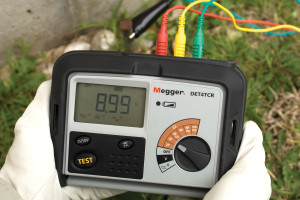Blog
August 2014
Let's Get Technical: Soil Resistivity Testing

Let's Get Technical: Soil Resistivity Testing

26 August 2014Soil resistivity testing is a standardized means of measuring and evaluating soil for its electrical properties. It is most useful in grounding, as a means of gathering data that is used in designing an effective ground electrode. This provides a valuable alternative to the drive-and-test method where ground rods are coupled and driven deeper, or added to a grid structure, and tested for resistance after each implementation until the required or specified resistance is achieved. Resistivity measurements can be entered into calculations along with the desired ground resistance and the complete electrode thereby designed before installation begins.

The most commonly used and familiar resistivity technique is the Wenner Method, named for Frank Wenner, a US Geological Survey engineer who defined it in 1915. Using a four-terminal ground tester, four equally spaced test probes are arranged in the order current-potential-potential-current, a Kelvin bridge configuration. Current is injected across the outer probes and voltage drop caused by the soil is measured across the two inner probes. A measurement is taken. The formula upon which the subsequent calculation rests is considerably complex, but fortunately, by Wenner spacing, it simplifies down to the familiar 2πaR, where R is the reading from the tester and a is the probe spacing. The result is expressed in units of ohm-centimeters (or meters, feet, etc), a volumetric measurement. This is average soil resistivity to a depth equal to the probe spacings.

With so simple a method, why would anyone want to use something else? Another method that offers some distinctive advantages is the Schlumberger-Palmer Method. Instead of equal spacing, Schlumberger-Palmer still uses four probes but has the potential probes set relatively close, with a much greater space to the current probes on the outside. The formula for calculation is now ρ = π(c + d)R/d. In this formula, b is the depth the probes are driven, c is the spacing between current and potential probes, and d is the spacing between the potentials, where c > 2d.

So what? Schlumberger-Palmer was devised in response to relatively large probe spacings, where the potential decreases rapidly in magnitude between the potential probes so that the measuring circuit doesn’t meet its level of sensitivity. (Remember, until recently, test instruments could be relatively crude. Modern microelectronic circuits are much improved in this regard.) The closer probe settings assured enough resistance to generate measureable voltage. (Remember also that test current spreads in a wide pattern through the earth. Don’t think of it as a straight-line path as in a wire.)But Schlumberger also adds the advantage of requiring fewer probe shifts. Whereas Wenner requires all four probes to be moved in order to measure at greater depth, only the outer probes are moved with Schlumberger, considerably reducing test time. The depth measured is equivalent to the distance from the center of the test rig to the outer probe, and thereby increases as the current probes are spread farther apart.

A third method, electrical coring, uses a vertical approach instead of spreading probes out over the surface. In this configuration, one current probe is driven in at the surface while the other is a spherical electrode suspended down a bore hole filled with liquid mud for good contact. The potential probes are also spherical electrodes, of smaller diameter, and suspended down the hole. The distance between the surface probe and the shallower potential probe is kept at six times the distance from the other potential to the current electrode deep in the bore hole. With this spacing, the formula is ρ = 4π(r1r2/r1-r2)R.

Here, r1 is the distance between the upper potential electrode and the current electrode at the bottom of the bore hole while r2 is the distance between the lower potential electrode and the current electrode in the bore hole. R, of course, is the reading from the meter. This yields the resistivity at the upper portion of the bore hole. If the bottom of the bore hole is to be measured, then the current electrode and the upper of the two potentials are interchanged. The method has the advantage of looking for changes in resistivity with depth while not requiring a great deal of surface space.

In selecting an appropriate tester, it must be remembered that the tester must have four terminals. Ground resistance tests (as opposed to resistivity) can be performed with only three terminals, and some testers, to be more economical, have only three terminals. To perform soil resistivity tests, you must have a 4-terminal model. Megger® offers four models: DET4TD2, DET4TR2, DET4TC2, and DET4TCR2. These four models differ only in power source and the addition of clamp capability (not used for resistivity). Power sources are disposable (D) and rechargeable (R). The addition of current and voltage clamps (C) provide useful functions for resistance testing but are not applicable to resistivity measurements.